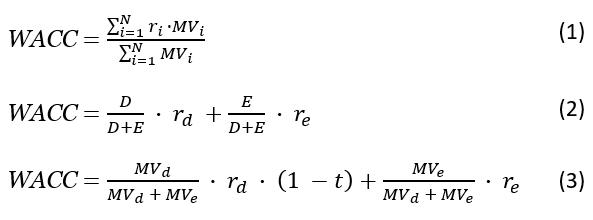# WACC Calculator

Use this online calculator to easily calculate the Weighted Average Cost of Capital (WACC) of a capital raise based on the cost of equity, cost of debt, and the corporate tax rate. WACC formula: generic, debt/equity and incorporating tax.

\$
%
\$
%
%
Share calculator:

Embed this tool:
get code

## Using the WACC calculator

Our online Weighted Average Cost of Capital calculator helps you easily calculate the cost of raising capital of any business. Simply enter the cost of raising capital through equity, debt, and the corporate tax the business operates under.

The calculator will then output the Weighted Average Cost of Capital, which is then often used as a discount rate for NPV calculations and discounted cash flow analysis.

## What is Weighted Average Cost of Capital?

In finance, WACC is defined as the rate a company expects to pay, on average, to all its security holders when financing its assets. This is why it is also called "cost of capital". The WACC of a company represents the minimum return it must earn on its assets to satisfy all its stockholders, creditors, owners, and other capital providers lest they flee.

In calculating it one takes into account the different sources of capital raised: common & preferred stock, straight, convertible or exchangeable debt, warrants, options, pension liabilities, subsidies, etc. Naturally, different securities are expected to result in different returns and WACC is useful since it weighs all capital components. The concept thus sees widespread use in financial management and investment management.

## How to calculate WACC?

So, how does one calculate weighted average cost of capital? For a simple calculation of this weighted mean one needs to know the different components of the cost of capital such as how much of it comes from equity or debt. Furthermore, the cost of each needs to be estimated. For equity this includes how much should be spent in order to maintain a share price that will satisfy investors. For debt the variable is the rate paid on outstanding debt. Finally, the applicable tax rate should be known.

Once accurate information is obtained, refer to the appropriate formula below or simply plug in the numbers in the WACC calculator above so it does the heavy lifting. After WACC is calculated, it can be used to compare a company's yields versus weighted average cost of capital to get an idea of how well it utilizes its capital assets. For example, let us say the company yield returns 22% and WACC is 10%. This means that the company is yielding 11% on every dollar it invests. It is creating 11 cents of value for each dollar of capital. However, if the yield is less than WACC, the company is destroying value and losing capital.

## WACC formula

There are several ways to write the formula for weighted average cost of capital. (1) below is the generic form wherein N is the number of sources of capital, ri is the required rate of return for security i and MVi is the market value of all outstanding securities i. (2) is the equation you can use if the only sources of financing are equity and debt with D being the total debt, E is the total shareholder's equity, Kd is the cost of debt and Ke is the equity cost.Formula (3) is the one used in this WACC formula calculator - it incorporates tax effects as well with t being the tax rate. Since there are many possible proxies for each element in the cost of capital formula there might be a fairly large range of defensible WACC analysis as result.

## Financial caution

This is a simple online tool which is a good starting point in estimating the average cost of a capital raise, but is by no means the end of such a process. You should always consult a qualified professional when making important financial decisions and long-term agreements, such as long-term bank deposits. Use the information provided by the weighted average cost of capital calculator critically and at your own risk.

#### Cite this calculator & page

If you'd like to cite this online calculator resource and information as provided on the page, you can use the following citation:
Georgiev G.Z., "WACC Calculator", [online] Available at: https://www.gigacalculator.com/calculators/wacc-calculator.php URL [Accessed Date: 01 Apr, 2023].